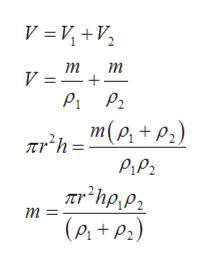# A solid cylinder has a radius of 0.05m and a height of 0.03m. The cylinder is composed of two different materials with materials with mass densities of 1950 kg/m3 and 1470 kg/m3. The cylinder contains an equal mass m of each of ther two materials, so that the total mass is M = 2m. What is the mass m? The volume of a cylinder is V = pie r2 h.

Question
21 views

A solid cylinder has a radius of 0.05m and a height of 0.03m. The cylinder is composed of two different materials with materials with mass densities of 1950 kg/mand 1470 kg/m3. The cylinder contains an equal mass m of each of ther two materials, so that the total mass is M = 2m. What is the mass m? The volume of a cylinder is V = pie rh.

check_circle

Step 1

Given,

Step 2

Density is defined as ratio of mass and volume. Since the mass of both materials are same so this situation can be expressed as below,

Step 3

As total volume of cylinder is fixed then mass of...help_outlineImage TranscriptioncloseV 3DИ, +V, т т V =" + m(p, + P2) Trh= Pipz Tr*hp,P2 т 3D (e,+P2) fullscreen

### Want to see the full answer?

See Solution

#### Want to see this answer and more?

Solutions are written by subject experts who are available 24/7. Questions are typically answered within 1 hour.*

See Solution
*Response times may vary by subject and question.
Tagged in

### Physics